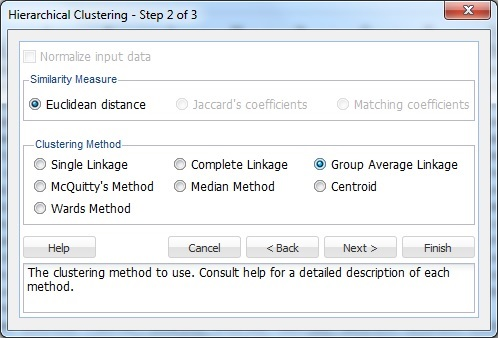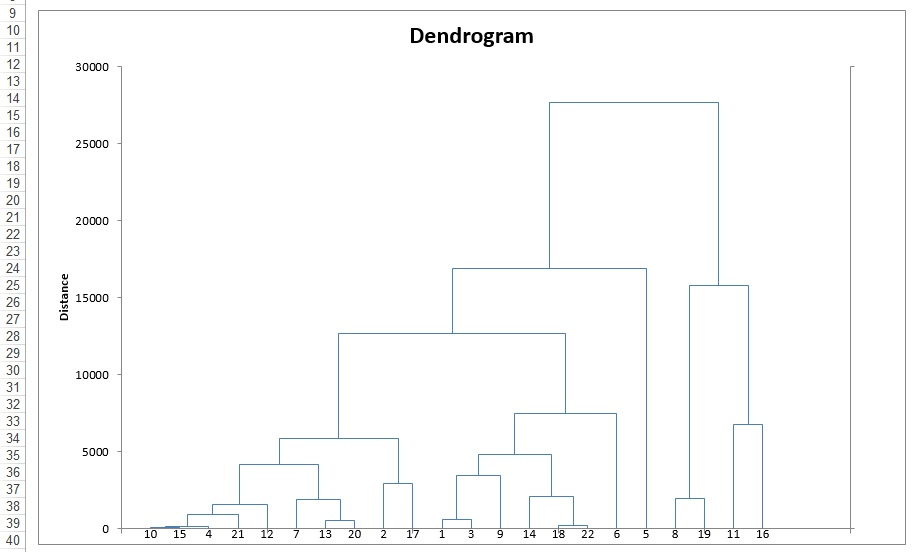The utilities.xlsx example data set (shown below) holds corporate data on 22 U.S. public utilities. This example illustrates how to use XLMiner to perform a cluster analysis using hierarchical clustering.

An example where clustering would be useful is a study to predict the cost impact of deregulation. To perform the requisite analysis, economists would be required to build a detailed cost model of the various utilities. It would save a considerable amount of time and effort by clustering similar types of utilities, building a detailed cost model for just one typical utility in each cluster, then scaling up from these models to estimate results for all utilities.

Each record includes eight observations. Before using a clustering technique, the data must be normalized or standardized. A popular method for normalizing continuous variables is to divide each variable by its standard deviation. After the variables are standardized, the distance can be computed between clusters using the Euclidean metric.

On the XLMiner ribbon, from the Applying Your Model tab, select Help - Examples, thenselect Forecasting/Data Mining Examples, and open the Utilities.xlsx example data set.Following is an explanation of the variables.

x1:  Fixed - charge covering ration (income/debt)

x2:  Rate of return of capital

x3:  Cost per KW capacity in place

x4:  Cost per KW capacity in place

x5:  Peak KWH demand growth from 1974 to 1975

x6:  Sales (KWH use per year)

x7:  Percent Nuclear

x8:  Total fuel costs (cents per KWH)

Select any cell in the data set, then on the XLMiner ribbon, from the Data Analysis tab, select Cluster - Hierarchical Clustering to open the Hierarchical Clustering dialog.

From the Variables in Input Data list, select variables x1 through x8, then click > to move the selected variables to the Selected Variables list.Click Next to advance to Step 2 of 3 dialog.Select Normalize input data to normalize the data by subtracting the variable's mean from each observation, and dividing by the standard deviation. Normalizing the data is important to ensure that the distance measure accords equal weight to each variable. Without normalization, the variable with the largest scale will dominate the measure.

Under Similarity Measure, Euclidean distance is selected by default. The Hierarchical Clustering method uses the Euclidean distance as the similarity measure for raw numeric data. When the data is binary, the remaining two options, Jaccard's coefficients and Matching coefficients, are enabled.

Under Clustering Method, select Group Average Linkage to calculate the average distance of all possible distances between each record in each cluster.

Click Next to open the Step 3 of 3 dialog.

Draw dendrogram and Show cluster membership are selected by default. At # Clusters, enter 4, then click Finish.XLMiner creates four clusters using the Group Average Linkage method. The output worksheet HC_Output is inserted immediately to the right of the Data worksheet.The top portion of this worksheet displays the choices made during the algorithm setup.

Further down the worksheet, the output details the history of the cluster formation. Initially, each individual case is considered its own cluster (single member in each cluster). XLMiner begins the method with # clusters = # cases. At stage 1, above, clusters (i.e., cases) 12 and 21 were found to be closer together than any other two clusters, so they are joined together into cluster 12. At this point, there is one cluster with two cases (cases 12 and 21), and 19 additional clusters that still have just one case in each. At stage 2, clusters 10 and 13 are found to be closer together than any other two clusters, so they are joined together into cluster 10.

This process continues until there is just one cluster. At various stages of the clustering process, there are different numbers of clusters. A dendrogram graph illustrates these steps.In the above dendrogram, the Sub-Cluster IDs are listed along the x-axis (in an order convenient for showing the cluster structure). The y-axis measures inter-cluster distance. Viewing cases 12 and 21, they have an inter-cluster distance of about 1.40. No other cases have a smaller inter-cluster distance, so 12 and 21 are joined into one cluster, indicated by the horizontal line linking them. Next, we see that cases 10 and 13 have the next smallest inter-cluster distance, so they are joined into one cluster. The next smallest inter-cluster distance is between clusters 4 and 20.If we draw a horizontal line through the diagram at any level on the y-axis (the distance measure), the vertical cluster lines that intersect the horizontal line indicate clusters whose members are at least that close to each other. If we draw a horizontal line at distance = 2.3, we see that there are 14 clusters. In addition, we can see that a case can belong to multiple clusters, depending on where we draw the line.

For purposes of assigning cases to clusters, we must specify the number of clusters in advance. In this example, we specified a limit of four.

When using Analytic Solver Pro or XLMIner Pro, if the number of training rows exceeds 30, then the dendrogram also displays Cluster Legends.

The HC_Clusters output worksheet includes the following table. This table displays the assignment of each record to the four clusters.The next example illustrates Hierarchical Clustering when the data represents the distance between the ith and jth records. When applied to raw data, Hierarchical Clustering converts the data into the distance matrix format before proceeding with the clustering algorithm. Providing the distance measures in the data, requires one less step for the Hierarchical Clustering algorithm.

Select a cell in the database, then on the XLMiner ribbon, from the Applying Your Model tab, select Help - Examples, then Forecasting/Data Mining Examples, to open the example file DistMatrix.xlsx. On the XLMiner ribbon, from the Data Analysis tab, select Cluster - Hierarchical Clustering to open the Hierarchical Clustering - Step 1 of 3 dialog.

Change the Data range to C3:X24, then at Data type, click the down arrow, and select Distance Matrix.All variables are added to the Input Variables list. Click Next to open the Step 2 of 3 dialog.

Notice Normalize input data, Jaccard's coefficients, and Matching coefficients are disabled when Distance Matrix is selected. Select Group Average Linkage as the Clustering Method, then click NextOn the Step 3 of 3 dialog, select Draw dendrogram (default) and Show cluster membership (default), then at # Clusters, enter 4. Click Finish.Output worksheets HC_Output1, HC_Clusters, and HC_Dendrogram1 are inserted immediately after Sheet1.

The Clustering Stages output (included on the HC_Output worksheet) is shown below.The Dendrogram output (included on the HC_Dendrogram worksheet) is shown below.Hierarchical Clustering is attractive to statisticians because it is not necessary to specify the number of clusters desired, and the clustering process can be easily illustrated with a dendrogram. However, the following are some limitations to Hierarchical Clustering.

Hierarchical Clustering requires computing and storing an n x n distance matrix. If using a large data set, this requirement can be very slow and require large amounts of memory.

The algorithm makes only one pass through the data set. As a result, records that are assigned erroneously will not be reassigned later in the process.

Clusters created through Hierarchical Clustering are not very stable. If records are eliminated, the results can be very different.

Outliers in the data can impact the results negatively.